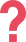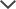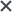## NNAT Kindergarten Sample Questions

Below there are NNAT Kindergarten sample questions followed by detailed explanations. Each question deals with a different subject that can be found on the actual test. Read the instructions for each question before you start solving them.

Pattern Completion

1. Look at the design in the large rectangle while paying attention to the missing square. Which answer choice completes the design?Imagine continuing the two curves across the empty square. The right side of the outermost curve will be white. The right side of the next curve will be yellow and the left side of the curve will be blue. The only answer choice that conforms to these requirements is answer choice D.

2. Look at the design in the large rectangle while paying attention to the missing square. Which answer choice completes the design?The correct answer is B. Look at the design in the large rectangle while paying attention to the missing square. Which answer choice completes the design?
In this design there are alternating blue and white waves. First notice the general color layout of what the empty square should be. The top and the bottom will be white while the center will be blue. Therefore, we can eliminate answer choices C, D, and E.
Imagine extending the curves of top and bottom lines of the middle curve through the empty square. Notice that both of these curved lines face the top of the empty square, and therefore we can eliminate answer choice A.  We are left with answer choice B, the correct answer.

3. Look at the design in the large rectangle while paying attention to the missing square. Which answer choice completes the design?The correct answer is C. Look at the design in the large rectangle while paying attention to the missing square. Which answer choice completes the pattern?

Imagine extending the curve of the circle across the empty square. The curve will extend across the top-right corner of the square. Answer choice C is the only answer choice which contains this property. We can eliminate answer choices A, B, D, and E because they contain lines which extend in different directions.

 Pattern Completion Summary: The NNAT Level A prep pack contains more than 150 Pattern Completion practice questions + an interactive video guide on this subject. All practice questions are accompanied by broad explanations that will ensure your child masters the Pattern Completions section of the NNAT Level A.

Reasoning by Analogy

1. In this kind of questions, there is a relation between the four boxes. The empty box must have the same relation as the others. You should examine across the rows or down the columns to figure out that relation. Do so in the direction in which the relation is clearer.The correct answer is A. Look at the top row. The left figure is the mirror image of the right figure, flipped horizontally. It is helpful to focus on a specific part of the shape. For example, in the left frame, the top blue square appears in the left corner and in the right frame, the top blue square appears in the right corner. Now, look at the bottom row. The figures in the bottom row will follow the same rules. The figure on the right will be the mirror image of the figure on the left. The left figure contains a white circle over four squares: yellow in the top-left and bottom-right corners, and blue in the opposite corners. The figure on the right will also contain a white circle over the four squares. The difference will be that the yellow squares will be positioned in the top-right and bottom-left corners, and the blue squares will be positioned in the top-left and bottom-right corners. The correct answer is answer choice A.

2. In this kind of questions, there is a relation between the four boxes. The empty box must have the same relation as the others. You should examine across the rows or down the columns to figure out that relation. Do so in the direction in which the relation is clearer.The correct answer is E. In the above analogy, look for a relationship between the figures across the rows and down the columns. The direction in which you examine the question should depend on where you can most easily visualize the analogous relationship.

The figures across the rows and down the columns are identical to each other. For example, look at the top row. The yellow square on the left is identical to the yellow square on the right. The shapes in the bottom row will exhibit the same relationship. Since the shape on the left is a yellow square, the shape on the right will also be a yellow square (answer choice E).

he top of the empty square, and therefore we can eliminate answer choice A.  We are left with answer choice B, the correct answer.

3. In this kind of questions, there is a relation between the four boxes. The empty box must have the same relation as the others. You should examine across the rows or down the columns to figure out that relation. Do so in the direction in which the relation is clearer.The correct answer is B. In the above analogy, look for a relationship between the figures across the rows and down the columns. The direction in which you examine the question should depend on where you can most easily visualize the analogous relationship.

In this analogy, the shapes remain the same across the rows, as does the color scheme going down the columns. The top row contains triangles and the bottom row contains pentagons. Thus, we can eliminate answer choices A and C, as they contain triangles rather than pentagons.

We are left with answer choices B, D, and E. The figures in the left column are yellow on a blue background and the figure in the right column is blue on a yellow background. Since the empty frame is in the right column, the figure will be blue on a yellow background. We can eliminate answer choice E, as  the figure is yellow on a blue background. Additionally, notice that the direction of the shapes does not change across the rows. Thus, we can eliminate answer choice D and are left with answer choice B, the correct answer.

 Reasoning by Analogy: The NNAT Level A prep pack contains 150+ Reasoning by Analogy practice questions + a Video tutorial on this subject. These practice questions, together with our detailed explanations and solutions, will help your child ace the Reasoning by Analogy section of the NNAT Level A.

Serial Reasoning

1. Look for a relationship between the figures across the rows and down the columns. The direction in which you examine the question should depend on where you can most easily visualize the analogous relationship.The correct answer is A. Across the top and middle rows in the above analogy, the figures rotate 180 degrees. The figures in the bottom row will adhere to the same property. Rotate the figure in the left frame 180 degrees to find the correct answer. Since the figure on the left contains a yellow arrow pointing downwards, and a white arrow pointing upwards, the answer choice will contain a yellow arrow pointing upwards and a white arrow pointing downwards (answer choice A).

2. Look for a relationship between the figures across the rows and down the columns. The direction in which you examine the question should depend on where you can most easily visualize the analogous relationship.The correct answer is D. In the above analogy, look for a relationship between the figures across the rows and down the columns. The direction in which you examine the question should depend on where you can most easily visualize the analogous relationship.

In this analogy, the shapes across the rows remain the same. Going down the columns, the colors of the shapes remain the same. In the top row, the left triangle is identical to the right triangle, except that the left triangle is yellow and the right triangle is blue. In the middle row, the left pentagon is identical to the right pentagon, except that the left pentagon is yellow and the right pentagon is blue. The shapes in the bottom row will adhere to the same rules. Since the shape on the left is a yellow diamond, the shape on the right will be a blue diamond (answer choice D).

top of the empty square, and therefore we can eliminate answer choice A.  We are left with answer choice B, the correct answer.

3. Look for a relationship between the figures across the rows and down the columns. The direction in which you examine the question should depend on where you can most easily visualize the analogous relationship.The correct answer is E. In the above analogy, look for a relationship between the figures across the rows and down the columns. The direction in which you examine the question should depend on where you can most easily visualize the analogous relationship.

Look at the top and middle rows. The figures on the right are mirror images of the figures on the left, flipped horizontally. Now look at the bottom row. The figures in the bottom row will follow the same rules. The figure on the left will be the mirror image of the figure on the right, flipped horizontally.

Take a look at the bottom row. Flip the image in the left frame horizontally to reach the answer choice. Since the left figure contains a yellow triangle at the top-left part of the figure, the right figure will contain a yellow triangle at the top-right part of the figure (answer choice E).

 Serial Reasoning Summary: The NNAT Level A prep pack contains more than 150 Serial Reasoning practice questions + a video lesson on this topic. Our prep pack includes elaborate explanations and detailed study guides to make sure your child gains the confidence he needs for the Serial Reasoning section.

TestPrep-Online package offers:

• Level specific, full-length practice tests
• Hundreds of practice questions
• Study guides for children
• Parent Manuals

### Our Online Services

At TestPrep-Online, we offer test preparation services that are fully available online. This online format allows you to access our study materials at your convenience. Study with your child where you want and when you want, without worrying about the added hassles of expensive classes or private tutors. Our study materials are easy to use, child-friendly, and specifically designed to help your child succeed. Start practicing with TestPrep-Online today with our free sample test for a free glimpse of what our study packs have to offer.To continue with the NNAT Video Academy, purchase any NNAT Premium Pack.

The NNAT and other trademarks are the property of their respective trademark holders. None of the trademark holders are affiliated with TestPrep-Online or this website.

Need HelpNeed Help